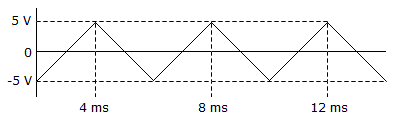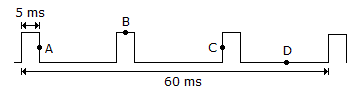# Electronics - Alternating Current and Voltage

### Exercise :: Alternating Current and Voltage - General Questions

6.

What is the instantaneous peak voltage at 250° on a 6 V peak sine wave?

 A. +5.64 V B. –5.64 V C. +26.13 V D. –26.13 V

Answer: Option B

Explanation:

No answer description available for this question. Let us discuss.

7.Calculate the positive-going slope of the waveform in the given circuit.

 A. 5 V/ms B. 2.5 V/ms C. 2.5 V/s D. 5 V/s

Answer: Option B

Explanation:

No answer description available for this question. Let us discuss.

8.

A rectangular wave that has a duty cycle of 50% could be called a

 A. "c" wave B. sawtooth wave C. square wave D. triangular wave

Answer: Option C

Explanation:

No answer description available for this question. Let us discuss.

9.Calculate the duty cycle of the repetitive pulse waveform in the given circuit.

 A. 8.33% B. 0% C. 33.33% D. 25%

Answer: Option D

Explanation:

No answer description available for this question. Let us discuss.

10.

The effective value of a sine wave is equal to

 A. 0.707 of peak voltage B. 0.636 of peak voltage C. sin 45° of peak voltage D. both 0.707 of peak voltage and sin 45° of peak voltage

Answer: Option D

Explanation:

No answer description available for this question. Let us discuss.

#### Current Affairs 2021

Interview Questions and Answers